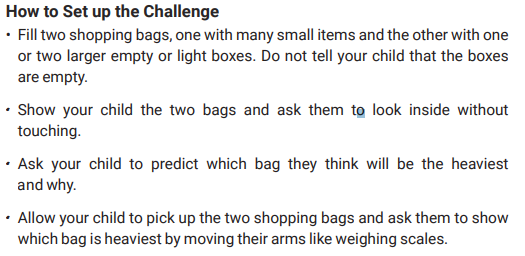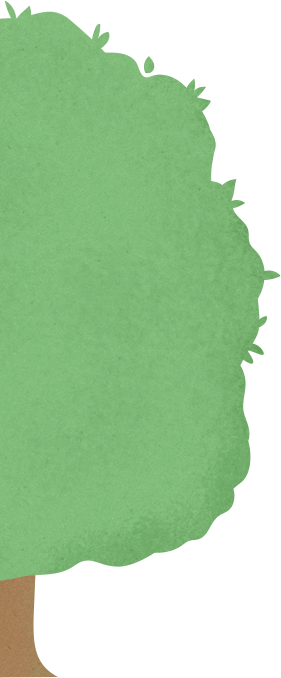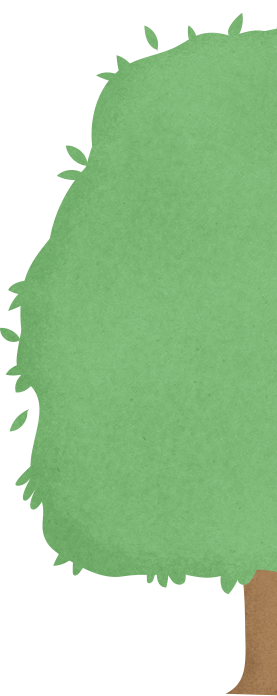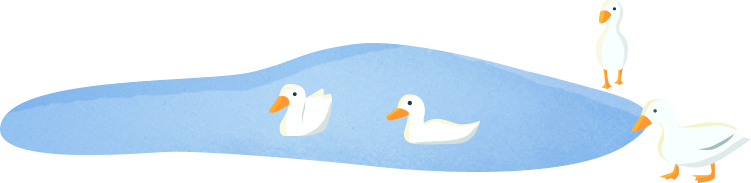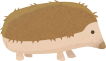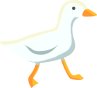## Maths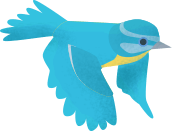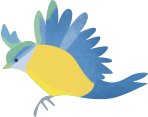# Maths

 Day 1 Activity: Number of the week The number of the week is a 2- digit number The number of the week is half of 30 The number of the week is 3 x 5 (5+5+5) The number of the week is one more than 14 The number of the week is one less than 16 The number of the week is 15 Activity: How many different ways can you make 15 e.g. 2+13 = 15, 5+10=15. Can you make up a worded problem using the number 15? Day 2 Activity: Can you guess the shape of the week? The shape of the week is a 2D shape The shape of the week has 8 sides. The shape of the week has 8 corners. The shape of the week is an octagon.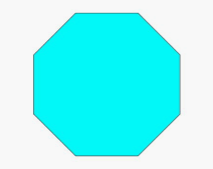Activity:                                                                      Can you make an Octagon handprint crab?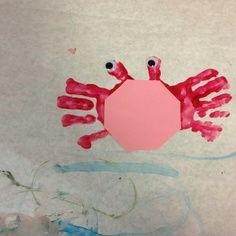Day 3 Weight Activity: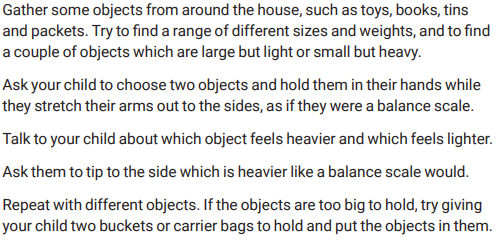Day 4 Weight Activity Make yourself a set of balancing scales with a coat hanger, string and two cups. Can you fill the cups with different objects – which side is heavier? How do you know? What happens to the scales?Day 5 Weight Activity: